# Resistivity

A. Resistance

All materials resist the flow of charges. The charges accelerate from one point of a circuit to another due to the potential difference between the two points. As the charges move from the negative to the positive terminals, they collide with the positive ions, which get in their way. Some of the kinetic energy is transferred due to the collisions, which results in electrical heating.

As the length of the conducting wire increases, so the resistance increases. The electrons have to go further through the material and the probability of collision also increases. The resistance of a conducting wire is directly proportional to its length.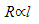Lets us consider a thicker wire. Its resistance will be less than that of a thin wire. This is because the charges have a bigger cross-sectional area to travel through, hence the probability of collision is less. The resistance of a wire is inversely proportional to its cross-sectional area.The resistance of a material also depends on the type of material. Metals are good conductors of electricity. Materials like wood and plastic are good insulators of electricity. A constant, ρ, is introduced in the equation of resistance. This constant is called resistivity. The value of resistivity is experimentally found out for every material at a particular temperature. The unit for resistance is ohm (Ω).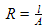B. Resistivity

The resistivity of a material is numerically equal to the resistance between opposite faces of a cube of the material, of unit length and unit cross-sectional area.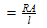The unit for resistivity is ohm metres (Ω m). The resistivity is highly dependent on temperature. The resistivity of metals increases with an increase in temperature, as shown in the table below. Collisions with the lattice atoms is the basic idea used to explain resistance.  When the electrons collide in the atoms in the fixed lattice, they bounce back and the overall movement becomes slower. When the temperature increases, the number of collisions increases and the resistance increases.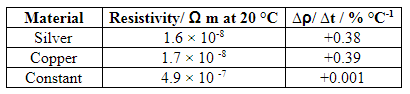The resistivity of a semiconductor decreases very rapidly with increasing temperature. The conduction in semiconductors is due to the movement of electrons and holes (positive charges) rather than only electrons, as in metals.

C. The Thermistor

The resistance of most of the metals increases to a certain extent with a rise in temperature. Some materials possess a negative temperature coefficient. These materials are made from semiconductor materials, usually oxides of metals. Components made from such materials are called thermistors. As the temperature increases, more conduction electrons are released, so the current increases and the resistance of a thermistor decreases with the increase in temperature.

Temperature and resistance variations of a thermistor are given in the table below.The data in the table above is represented in Figure 1 below. The variation is non-linear. Over a limited range of temperature, the variation is approximately exponential.Figure 1: Resistance Versus Temperature Graph of a Thermistor

D. Superconductivity

A superconductor is a perfect conductor. A closed loop of superconducting wire carrying a current will continue to carry the current as long as the temperature is kept below the critical temperature TC. This current is called a persistent current.

In 1912, Dutch physicist Kamerlingh Onnes discovered that mercury cooled to 4.15 K loses its resistivity completely and becomes a superconductor. Therefore, 4.15 k is the critical temperature for mercury.

Several others metals apart from mercury exhibit superconductivity. A few alloys also exhibit superconductivity. A few examples of superconductors and their critical temperatures are given in the table below.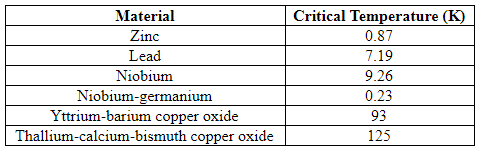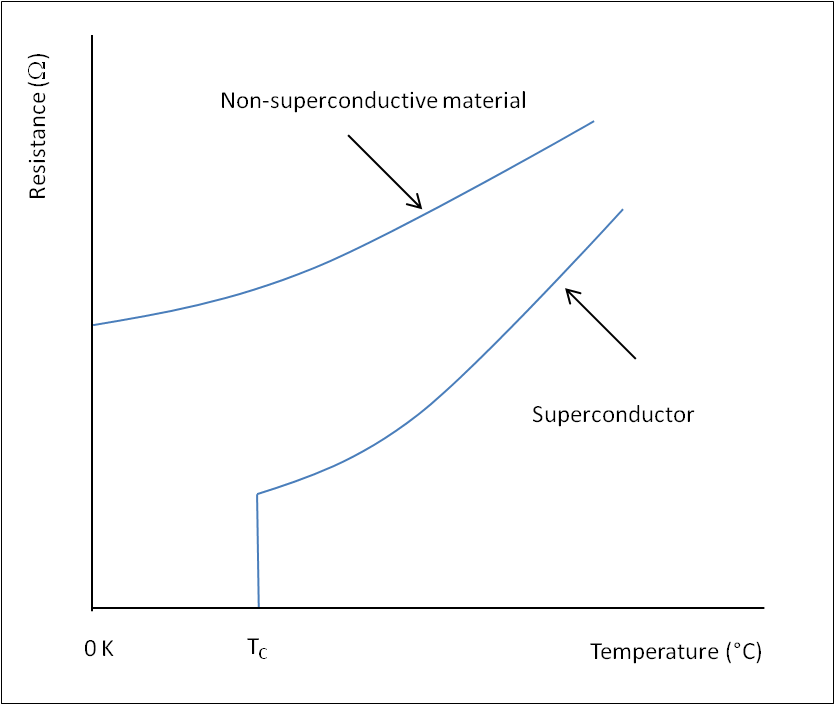Figure 2: Resistance Versus Temperature Graph of a Superconductor

The theory to explain the behaviour of superconductors was found in 1950. It requires energy of electrons to scatter as they move through the metal lattice. These scatterings are the collisions that lead to heating through resistance. At low temperatures, this energy is not available, so the electrons move freely with zero resistance.

Superconductors are used for various applications. They produce strong magnetic fields required for MRI scanning in medicine and to confine beams of particles in accelerators such as the Large Hadron Collider. Superconductors are used in SQUID (superconducting quantum interference device) magnetometers. SQUID can measure the fine magnetic fields associated with activity in the brain.

E. Summary

• Resistivity (ρ) of a material is the resistance between opposite faces of a unit cube of the material:• The resistance of a metal increases with increasing temperature.
• The resistance of a semiconductor decreases very rapidly with increasing temperature.
• The resistance of a thermistor varies with the temperature in a non-linear manner.
• Superconductors exhibit zero resistance when the temperature is less than the critical temperature.